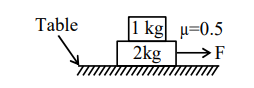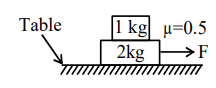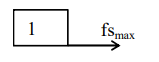Deepak Scored 45->99%ile with Bounce Back Crack Course. You can do it too!

# Solve this following

Question:

The coefficient of static friction between two blocks is $0.5$ and the table is smooth. The maximum horizontal force that can be applied to move the blocks together is .......N.

$\left(\right.$ take $\left.\mathrm{g}=10 \mathrm{~ms}^{-2}\right)$Solution:$\mathrm{F}=3 \mathrm{a}($ For system $)$     ........................(I)$\mathrm{fs}_{\max }=1 \mathrm{a}($ for $1 \mathrm{~kg}$ block) ..................(II)

$\mu \times 1 \times g=a$

$\Rightarrow 5=\mathrm{a}$

$\mathrm{F}=15 \mathrm{~N}$